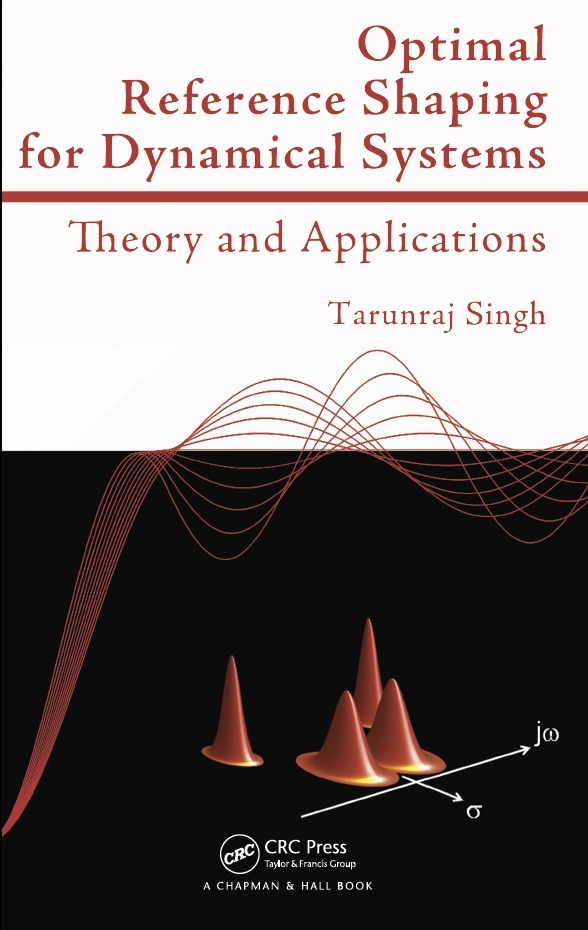Optimal Reference Shaping for Dynamical Systems: Theory and Applications Optimal Reference Shaping for Dynamical Systems: Theory and Applications provides a rigorous yet accessible presentation of the theory and numerical techniques used to shape control system inputs for achieving precise control when modeling uncertainties exist. It includes up-to-date techniques for the design of command-shaped profiles for precise, robust, and rapid point-to-point control of underdamped systems. After developing models for applications in which the dynamics are dominated by lightly damped modes, the book describes the time-delay (input shaper) design techniques and reviews calculus of variations. It then focuses on four control problems: time-optimal, fuel/time-optimal, fuel limited time-optimal, and jerk liimited time-optimal control. The following two chapters deal with the minimax formulation for the design of robust time-delay filters and the design of input-constrained control profiles for point-to-point maneuvers of systems subject to friction. The final chapter presents numerical techniques for solving the problem of design of input shapers. Features: Presents the frequency domain approach for designing input-shaper/time-delay filter Illustrates the design of optimal command shapers through gradient-based and convex programming-based approaches Covers the minimax design of robust command shapers and state feedback controllers Discusses the control of vibratory systems that are subject to Coulomb friction Includes many examples problems that represent actual engineering systemsMatlab Code

Chapter 2
Chapter 3

Chapter 7
• Example_71.m - Time-Optimal Control of Benchmark Problem
• Example_72.m - Time-Delay Filters for Single-Mode System
• Example_73.m - L_infty Norm based Minimax Time-Delay Filters for Single-Mode System
• Example_74.m - L_infty Norm based Minimax Time-Delay Filters for Benchmark Problem
• Example_75.m - L_1 Norm based Minimax Time-Delay Filters for Single-Mode System
• Example_76.m - L_1 Norm based Minimax Time-Delay Filters for Benchmark Problem

Pendulum Control

Step Input Response Shaped Step Input Response Arbitrary Input Response Shaped Arbitrary Input Response